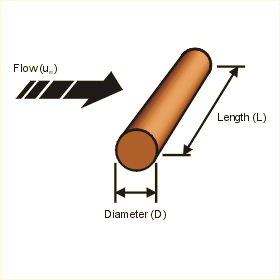Close

This page calculates the average heat transfer coefficient and rod temperature for an isothermal (constant temperature) circular rod in free stream flow. The convection calculation automatically switches between laminar and turbulent convection correlations based on Reynolds number.The calculation is based on Nusselt number correlations.

The heat flow (q) from the plate is calculated as:

q = h A x (Tp - Ta)

Where h is the average heat transfer coefficient, A is the area of the rod, Tr is the rod temperature and Ta is the ambient fluid temperature. h is defined as:

h = Nu k / D

Where Nu is the Nusselt Number, k the conductivity of the fluid and D the diameter of the rod. The Nusselt number is calculated as:

Nu = C Ren Pr0.33

Where Re is the Reynolds number, Pr is the Prandtl number, and C and n are variables that change with the value of the Reynolds number.

 Re C n 0.4 - 4 0.989 0.330 4 - 40 0.911 0.385 40 - 4000 0.683 0.466 4000 - 40000 0.193 0.618 40000 - 400000 0.0266 0.805

The Reynolds number and Prandlt number are calculated using fluid properties as follows:

Pr = kinematic viscosity / thermal diffusivity
Re = fluid velocity x diameter / kinematic viscosity

In addition, you must define the fluid properties at the film temperature Tf defined as follows:

Tf = (Tr + Ta) / 2

The above correlations are valid for Reynolds numbers in the range of 0.4 and 400000 and Prandlt numbers in the range of 0.6 and 50. These calculations are not suitable for low Prandtl fluids like liquid metals and high Prandtl fluids like heavy oils or silicons.

References

Holman, J.P., Heat Transfer, 7th ed., McGraw Hill Book Company, New York, 1990. p 281 - 303